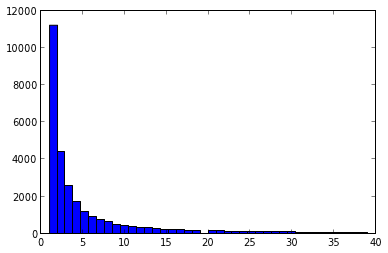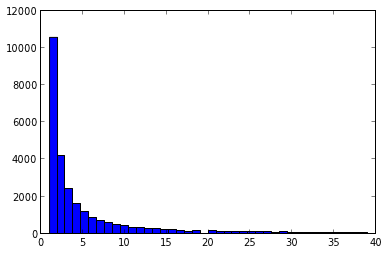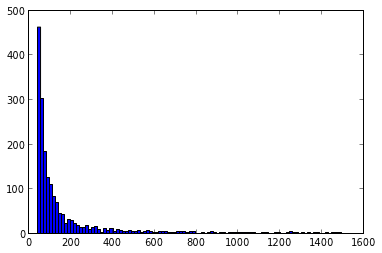In :
import nltk
from nltk.corpus import movie_reviews

In :
negids = movie_reviews.fileids('neg')
posids = movie_reviews.fileids('pos')

In :
#before creating test and training sets, first survey full corpus
negwords=[]
for file in negids:
negwords += [w.lower() for w in movie_reviews.words(file) if w.isalpha()]

In :
poswords=[]
for file in posids:
poswords += [w.lower() for w in movie_reviews.words(file) if w.isalpha()]

In :
len(negwords),len(poswords)

Out:
(628315, 701729)
In :
fdistneg= nltk.FreqDist(negwords)
fdistpos= nltk.FreqDist(poswords)

In :
fdistneg.items()[:10],'...',fdistneg.items()[-10:]

Out:
([('the', 35058),
('a', 17910),
('and', 15680),
('of', 15487),
('to', 15420),
('is', 11136),
('in', 10097),
('s', 8854),
('that', 7803),
('it', 7756)],
'...',
[('zinger', 1),
('zipper', 1),
('zippy', 1),
('zoe', 1),
('zombified', 1),
('zoologists', 1),
('zsigmond', 1),
('zulu', 1),
('zwigoff', 1),
('zzzzzzz', 1)])
In :
fdistpos.items()[:10],'...',fdistpos.items()[-10:]

Out:
([('the', 41471),
('a', 20196),
('and', 19896),
('of', 18636),
('to', 16517),
('is', 14059),
('in', 11725),
('s', 9659),
('it', 8351),
('that', 8121)],
'...',
[('zones', 1),
('zookeeper', 1),
('zookeepers', 1),
('zoologist', 1),
('zophres', 1),
('zukovsky', 1),
('zurg', 1),
('zus', 1),
('zweibel', 1),
('zwigoff', 1)])
In :
n, bins, patches = hist([v for v in fdistpos.values() if v <40],40)
#over 11,000 words appear only once in positive corpusIn :
n, bins, patches = hist([v for v in fdistneg.values() if v <40],40)
#over 10,000 words appear only once in negative corpusIn :
n, bins, patches = hist([v for v in fdistpos.values() if v >=40 and v<1500],100)
#mainly stopwords about 1500In :
#this is approach from http://nltk.org/book/ch06.html  (examples 6.4, 6.5)
all_words = nltk.FreqDist(w.lower() for w in movie_reviews.words())
word_features = all_words.keys()[:2000]

In :
def document_features(document):
document_words = set(document)  #doesn't use #occurrences
features = {}
for word in word_features:
features['contains(%s)' % word] = (word in document_words)
return features

In :
#have a look at features for just one document
x=document_features(movie_reviews.words('pos/cv957_8737.txt'))
[(k,v) for k,v in x.items()][:10]

Out:
[('contains(waste)', False),
('contains(lot)', False),
('contains(*)', True),
('contains(black)', False),
('contains(rated)', False),
('contains(potential)', False),
('contains(m)', False),
('contains(understand)', False),
('contains(drug)', True),
('contains(case)', False)]
In :
#this pairs the words in each doc, with the pos/neg category
documents = [(list(movie_reviews.words(fileid)), category)
for category in movie_reviews.categories()
for fileid in movie_reviews.fileids(category)]
random.shuffle(documents)

In :
#this pairs the above features for each doc with the pos/neg category
featuresets = [(document_features(d), c) for (d,c) in documents]

In :
train_set, test_set = featuresets[100:], featuresets[:100] # test on first 100

In :
classifier = nltk.NaiveBayesClassifier.train(train_set)

In :
nltk.classify.accuracy(classifier, test_set)

Out:
0.77
In :
classifier.show_most_informative_features(5)

Most Informative Features
contains(outstanding) = True              pos : neg    =     11.0 : 1.0
contains(mulan) = True              pos : neg    =      8.5 : 1.0
contains(seagal) = True              neg : pos    =      8.1 : 1.0
contains(wonderfully) = True              pos : neg    =      6.7 : 1.0
contains(damon) = True              pos : neg    =      5.8 : 1.0

In :
#Now try it using #appearances, not just binary true/false
random.shuffle(negids)  #shuffle both,
random.shuffle(posids)   #to pull at random from two sets

In :
negwords=[] #use last 950 as training set
for filename in negids[50:]:
negwords += [w.lower() for w in movie_reviews.words(filename) if w.isalpha()]
poswords=[] #use last 950 as training set
for filename in posids[50:]:
poswords += [w.lower() for w in movie_reviews.words(filename) if w.isalpha()]

In :
colfreqneg= nltk.FreqDist(negwords)  #training sets
colfreqpos= nltk.FreqDist(poswords)

In :
colfreq=nltk.FreqDist(negwords+poswords)  #full distribution
len(colfreq)  #size of full vocab

Out:
38278
In :
colfreq.items()[:10],'...',colfreq.items()[40:50],'...',colfreq.items()[-10:]  #survey what's there

Out:
([('the', 72745),
('a', 36225),
('and', 33898),
('of', 32476),
('to', 30368),
('is', 23965),
('in', 20787),
('s', 17619),
('it', 15322),
('that', 15094)],
'...',
[('so', 3481),
('out', 3471),
('up', 3227),
('more', 3194),
('what', 3135),
('when', 3111),
('which', 3021),
('their', 2979),
('or', 2971)],
'...',
[('zoologists', 1),
('zophres', 1),
('zorg', 1),
('zukovsky', 1),
('zulu', 1),
('zurg', 1),
('zus', 1),
('zweibel', 1),
('zwigoff', 1),
('zzzzzzz', 1)])
In :
vocab = [w for w,v in colfreq.items() if v >=10 and v < 2950]  #use frequency cutoffs
len(vocab)  #reduces vocabulary

Out:
8913
In :
len([w for w,v in colfreq.items() if v ==10]) #items that occur ten times

Out:
601
In :
Nneg=sum([v for w,v in colfreqneg.items() if w in vocab])
Npos=sum([v for w,v in colfreqpos.items() if w in vocab])
Nneg,Npos  #total numbers of words in neb and pos training sets

Out:
(312064, 349559)
In :
# and now train the weights
pweight = {}    # log ( p(w|P)/p(w|N) )
lc=log(float(Nneg)/Npos)
for w in vocab:   #need some "smoothing" to avoid any zeroes
if colfreqpos[w] == 0:
r=1./colfreqneg[w]
elif colfreqneg[w] == 0:
r= float(colfreqpos[w])
else:
r=float(colfreqpos[w])/colfreqneg[w]
pweight[w] = log(r) + lc

In :
sw=sorted(pweight.keys(),key=pweight.get) #sort to have a look

In :
[(w,pweight[w]) for w in sw[:10]]

Out:
[('nbsp', -4.1739070755837586),
('seagal', -3.7243819776815639),
('jawbreaker', -3.514661446699495),
('webb', -3.514661446699495),
('magoo', -3.4456685752125433),
('hudson', -3.4456685752125433),
('sphere', -3.4093009310416686),
('jakob', -3.4093009310416686),
('brenner', -3.3323398899055401),
('heckerling', -3.2045065183956551)]
In :
[(w,pweight[w]) for w in sw[-10:]]

Out:
[('leila', 3.3205231394478067),
('homer', 3.3205231394478067),
('guido', 3.352271837762387),
('gattaca', 3.441883996452074),
('argento', 3.4700548734187704),
('ordell', 3.7783562330732869),
('lebowski', 3.8938691201951317),
('shrek', 4.0296706613541931),
('flynt', 4.255983787429682),
('mulan', 4.3524440536172442)]
In :
wrong=[]
for filename in negids[:50]:
score=0  #calculate the score by summing the weights
for w in movie_reviews.words(filename):
if w.lower() in pweight: score += pweight[w]
if score >0: wrong.append((filename,score))

In :
for filename in posids[:50]:
score=0  #calculate the score by summing the weights
for w in movie_reviews.words(filename):
if w.lower() in pweight: score += pweight[w]
if score <0: wrong.append((filename,score))

In :
len(wrong)

Out:
23
In :
#23/100 wrong so strangely enough, the same 77% as before, which ones?
wrong

Out:
[('neg/cv646_16817.txt', 18.976833153912455),
('neg/cv845_15886.txt', 10.856734309834804),
('neg/cv826_12761.txt', 10.69995724084629),
('neg/cv472_29140.txt', 62.275057730932971),
('neg/cv042_11927.txt', 20.254416860549032),
('neg/cv954_19932.txt', 3.0406699227259919),
('neg/cv223_28923.txt', 24.677843780221501),
('neg/cv232_16768.txt', 23.291006113345841),
('neg/cv524_24885.txt', 18.17507963356196),
('neg/cv445_26683.txt', 1.0346272858658174),
('neg/cv571_29292.txt', 53.25739555277061),
('pos/cv889_21430.txt', -16.747308980341252),
('pos/cv149_15670.txt', -32.725408184935539),
('pos/cv578_15094.txt', -27.132098470382942),
('pos/cv610_2287.txt', -1.3282123066582423),
('pos/cv828_19831.txt', -3.2098413450969772),
('pos/cv876_9390.txt', -18.637489724888308),
('pos/cv792_3832.txt', -38.404088586164221),
('pos/cv082_11080.txt', -14.289811479316835),
('pos/cv380_7574.txt', -18.423949352346391),
('pos/cv964_6021.txt', -51.870853413891943),
('pos/cv464_15650.txt', -5.7247161687226891),
('pos/cv077_22138.txt', -3.2137203260856642)]
In :
#Now try it again, but instead of collection frequency cutoff,
# use doc frequency, i.e., appear in minimum number of documents
# rather then minimum number of times overall.
#   using set() will count only once per document:
negdocwords=[] #use last 950 as training set
for filename in negids[50:]:
negdocwords += set([w.lower() for w in movie_reviews.words(filename) if w.isalpha()])
posdocwords=[] #use last 950 as training set
for filename in posids[50:]:
posdocwords += set([w.lower() for w in movie_reviews.words(filename) if w.isalpha()])

In :
len(negwords),len(poswords),len(negdocwords),len(posdocwords)
#removing multiplicity reduces overall numbers

Out:
(600238, 664761, 308806, 330274)
In :
docfreq=nltk.FreqDist(negdocwords+posdocwords)  #full distribution
len(docfreq)  #size of full vocab

Out:
38278
In :
docfreq.items()[:10]

Out:
[('the', 1900),
('of', 1899),
('and', 1898),
('to', 1898),
('a', 1897),
('in', 1896),
('is', 1895),
('it', 1867),
('s', 1866),
('that', 1866)]
In :
vocab = [w for w,v in docfreq.items() if v >= 10 and v < 1900*.75]
#use frequency cutoffs, must appear in at least 10 docs, but not in more than 3/4 of them
len(vocab)

Out:
7793
In :
#with vocab now chosen, determine overall number of terms in neg and pos
Nneg=sum([v for w,v in colfreqneg.items() if w in vocab])
Npos=sum([v for w,v in colfreqpos.items() if w in vocab])
print Nneg,Npos  #total numbers of words in neb and pos training sets

# and now train the weights
#(same as above, use colfreq for weights, only used doc frequency to select vocab)
pweight = {}    # log ( p(w|P)/p(w|N) )
lc=log(float(Nneg)/Npos)
for w in vocab:   #need some "smoothing" to avoid zeros
if colfreqpos[w] == 0:
r=1./colfreqneg[w]
elif colfreqneg[w] == 0:
r= float(colfreqpos[w])
else:
r=float(colfreqpos[w])/colfreqneg[w]
pweight[w] = log(r) + lc

337577 367912

In :
sw=sorted(pweight.keys(),key=pweight.get) #sort to have a look
[(w,pweight[w]) for w in sw[:10]]
#note that nbsp is gone

Out:
[('seagal', -3.6969680593432295),
('hudson', -3.4182546568742089),
('silverstone', -3.0304891258654458),
('schumacher', -2.9764219045951701),
('fares', -2.9764219045951701),
('degenerates', -2.7941003478012152),
('incoherent', -2.7941003478012152),
('illogical', -2.725107476314264),
('alicia', -2.725107476314264)]
In :
[(w,pweight[w]) for w in sw[-10:]]

Out:
[('frances', 2.747163197357211),
('regard', 2.747163197357211),
('damon', 2.7617619967783638),
('avoids', 2.8043216111971594),
('turturro', 2.8043216111971594),
('astounding', 2.8043216111971594),
('hatred', 2.8583888324674351),
('gattaca', 3.0920036836489406),
('winslet', 3.2461543634761987),
('mulan', 4.3447666521443082)]
In :
#look at some document frequencies
docfreq['mulan'],docfreq['winslet'],docfreq['damon'],docfreq['seagal']

Out:
(13, 13, 32, 22)
In :
wrong=[]
for filename in negids[:50]:
score=0  #calculate the score by summing the weights
for w in movie_reviews.words(filename):
if w.lower() in pweight: score += pweight[w]
if score >0: wrong.append((filename,score))
for filename in posids[:50]:
score=0  #calculate the score by summing the weights
for w in movie_reviews.words(filename):
if w.lower() in pweight: score += pweight[w]
if score <0: wrong.append((filename,score))

In :
len(wrong)   # now up to 86%

Out:
14
In :
# improved accuracy shows importance of feature selection,
# though should really check for various randomly selected test sets
wrong

Out:
[('neg/cv010_29063.txt', 8.7813269632053945),
('neg/cv147_22625.txt', 9.2600147604274969),
('neg/cv162_10977.txt', 2.1956587216326313),
('neg/cv700_23163.txt', 17.863280457044834),
('neg/cv024_7033.txt', 26.649670583665813),
('neg/cv091_7899.txt', 8.0726724627755662),
('neg/cv889_22670.txt', 7.3758350657541261),
('neg/cv735_20218.txt', 1.1416418117950169),
('pos/cv636_15279.txt', -13.43445748911274),
('pos/cv489_17906.txt', -23.447681704857278),
('pos/cv952_25240.txt', -26.003954959670907),
('pos/cv230_7428.txt', -8.6124344308240168),
('pos/cv464_15650.txt', -2.6967653788762509),
('pos/cv685_5947.txt', -8.8190914867265739)]
In :
#so try 20-fold cross validation
#break it into 20 blocks, omit the i'th and use as test set
negwords=[[] for i in range(20)]
poswords=[[] for i in range(20)]
negdocwords=[[] for i in range(20)]
posdocwords=[[] for i in range(20)]
for i in range(20):
for k in range(20):
if k==i: continue #skip the i'th block of 50 files
for filename in negids[50*k:50*(k+1)]:
negwords[i] += [w.lower() for w in movie_reviews.words(filename) if w.isalpha()]
negdocwords[i] += set([w.lower() for w in movie_reviews.words(filename) if w.isalpha()])
for filename in posids[50*k:50*(k+1)]:
poswords[i] += [w.lower() for w in movie_reviews.words(filename) if w.isalpha()]
posdocwords[i] += set([w.lower() for w in movie_reviews.words(filename) if w.isalpha()])

In :
#now same as before, just make lists to do it 20 times
docfreq=[]
colfreqneg=[]
colfreqpos=[]
vocab = []

for i in range(20):
docfreq.append(nltk.FreqDist(negdocwords[i]+posdocwords[i]))  #full distributions

colfreqneg.append(nltk.FreqDist(negwords[i]))  #training sets
colfreqpos.append(nltk.FreqDist(poswords[i]))
#use frequency cutoffs, must appear in at least 10 docs, but not in more than 3/4 of them
vocab.append([w for w,v in docfreq[i].items() if v >= 10 and v < 1900*.75])

In :
#calculate 20 sets of weights
pweight = [{} for i in range(20)]    # log ( p(w|P)/p(w|N) )
lc=log(float(Nneg)/Npos)
for i in range(20):
for w in vocab[i]:   #need some "smoothing" to avoid zeros
if colfreqpos[i][w] == 0:
r=1./colfreqneg[i][w]
elif colfreqneg[i][w] == 0:
r= float(colfreqpos[i][w])
else:
r=float(colfreqpos[i][w])/colfreqneg[i][w]
pweight[i][w] = log(r) + lc

In :
#collect the number of wrongs for each of the 20 cross-validations
wrong=[[] for i in range(20)]
for i in range(20):
for filename in negids[50*i:50*(i+1)]:
score=0  #calculate the score by summing the weights
for w in movie_reviews.words(filename):
if w.lower() in pweight[i]: score += pweight[i][w]
if score >0: wrong[i].append((filename,score))
for filename in posids[50*i:50*(i+1)]:
score=0  #calculate the score by summing the weights
for w in movie_reviews.words(filename):
if w.lower() in pweight[i]: score += pweight[i][w]
if score <0: wrong[i].append((filename,score))

In :
print map(len,wrong)
print mean(map(len,wrong))

[14, 15, 22, 24, 20, 11, 16, 16, 14, 11, 19, 23, 21, 14, 20, 17, 14, 8, 21, 17]
16.85

In [ ]: